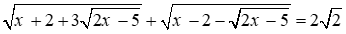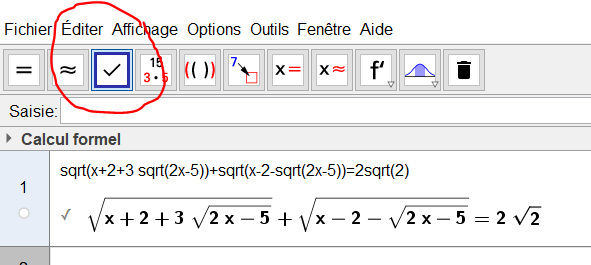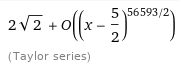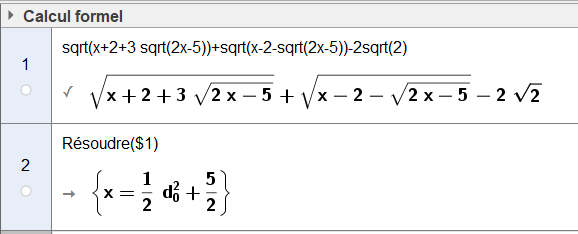# équation

barakatomar1960 shared this problem 2 years ago
Not a Problem

Bonjour tout le monde

Dans Calcul formel

Quand je veux écrire√(x+2+3√(2x-5))+√(x-2-√(2x-5))=2√(2)

Geogebra affiche 2√(2)=2√(2)

Merci d'avance1

Bonsoir, je confirme si on ne choisit pas le menu "Conserver la saisie" sinon c'est OK1

if you graph sqrt(x+2+3 sqrt(2x-5))+sqrt(x-2-sqrt(2x-5)) you can see that the equation have an interval of solutions [2.5,3] with the machine precision

GG has not implemented equations with infinite number of solutions

wolfram alpha calculatesso for a computer the function gives 2 sqrt(2) in interval

in fact, it is not possible to program algebraic solutions for all equations

https://www.wolframalpha.co...1

CAS >1

what means